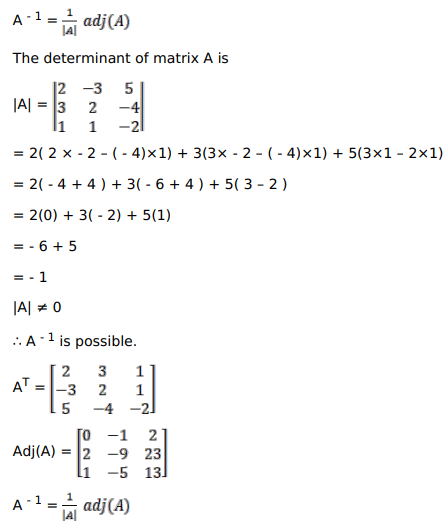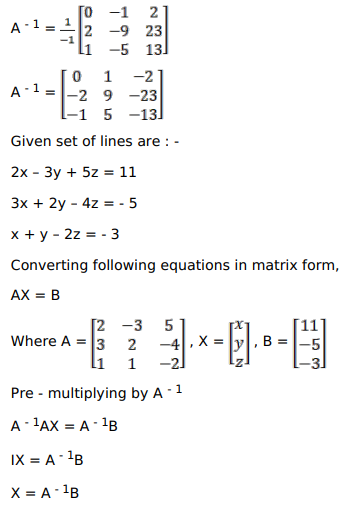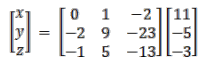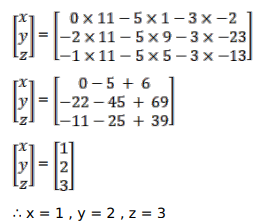# Solve this following

Question:

If $A=\left(\begin{array}{ccc}2 & -3 & 5 \\ 3 & 2 & -4 \\ 1 & 1 & -2\end{array}\right)$, find $A^{-1}$

Using $A^{-1}$, solve the following system of equations:

$2 x-3 y+5 z=11$

$3 x+2 y-4 z=-5$

$x+y-2 z=-3$

Solution:

Given,

$A=\left[\begin{array}{ccc}2 & -3 & 5 \\ 3 & 2 & -4 \\ 1 & 1 & -2\end{array}\right]$